# 100 100 200 200 300 300 400 400

• Slides: 49\$100 \$100 \$200 \$200 \$300 \$300 \$400 \$400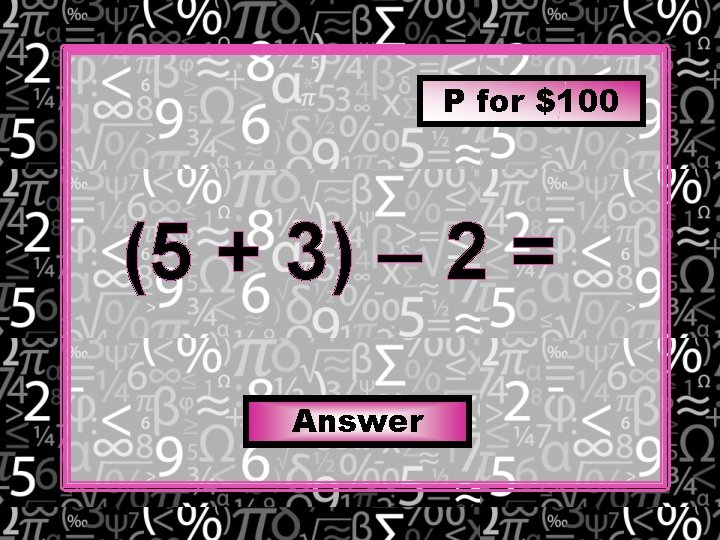P for \$100 (5 + 3) – 2 = Answer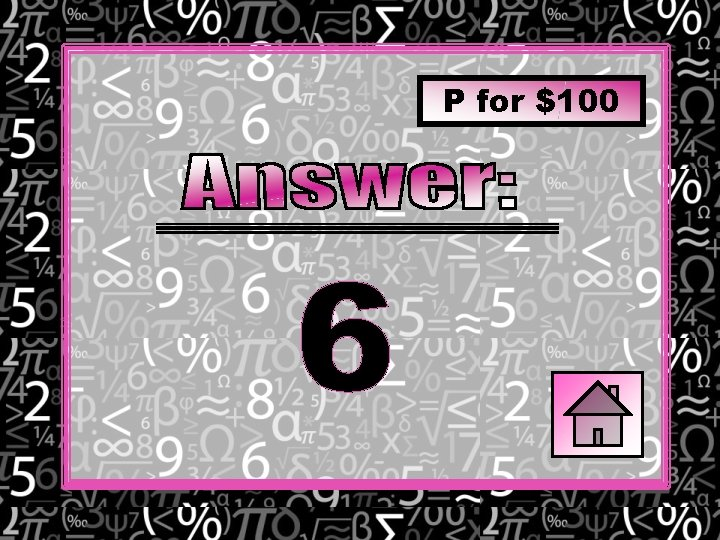P for \$100 6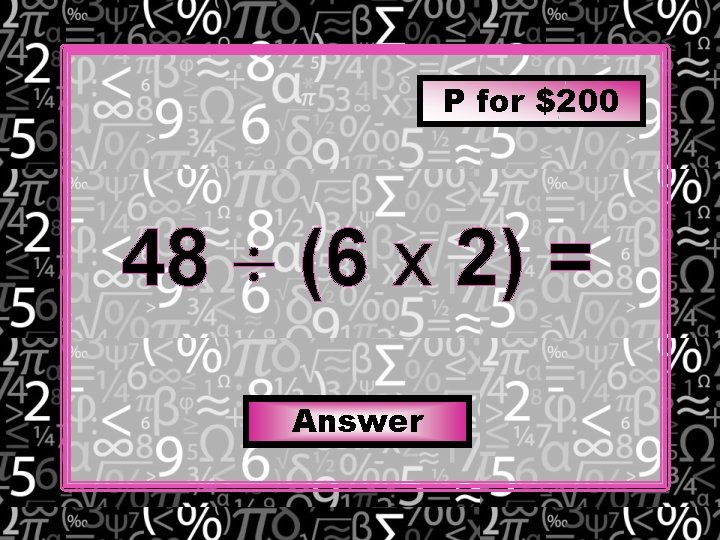P for \$200 48 (6 x 2) = Answer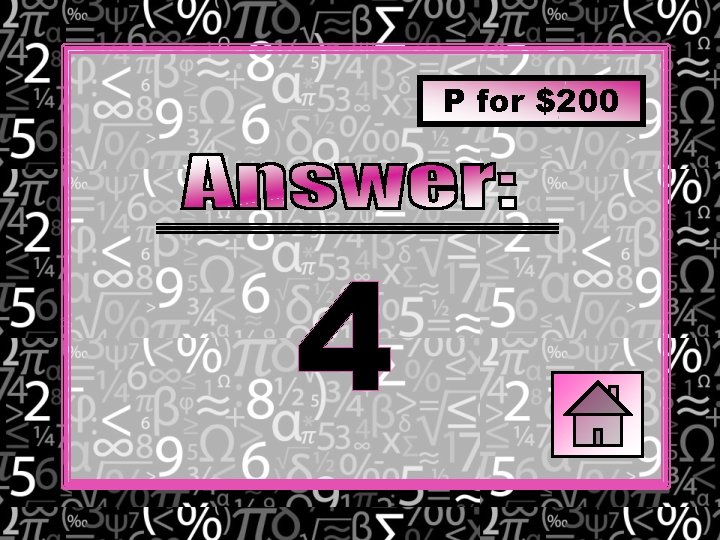P for \$200 4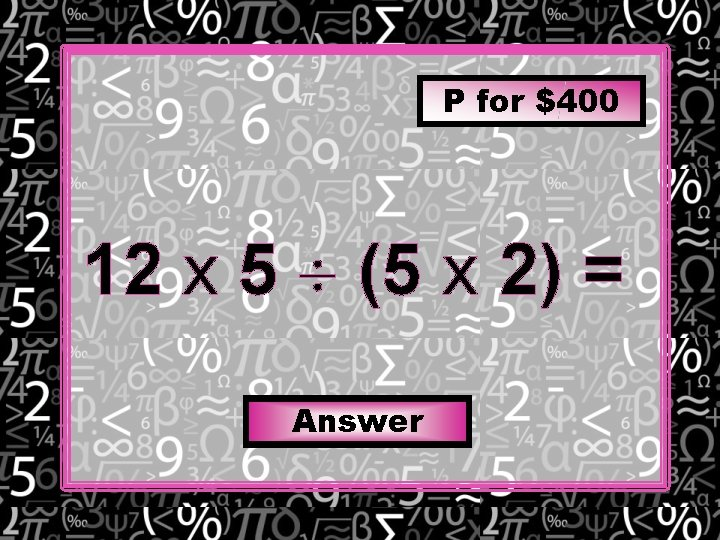P for \$400 12 x 5 (5 x 2) = Answer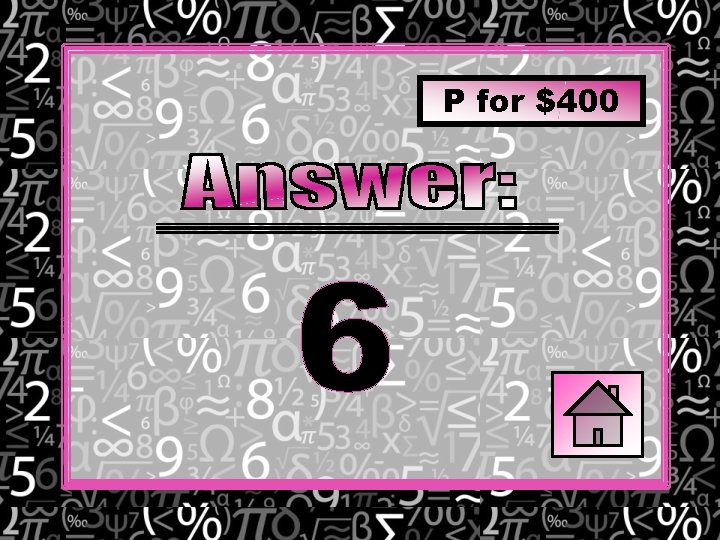P for \$400 6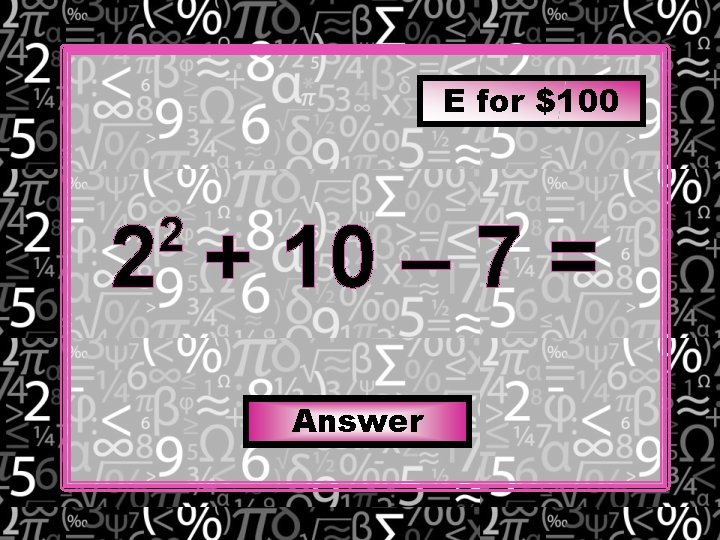E for \$100 2 + 10 – 7 = 2 AnswerE for \$100 7E for \$300 42 7 + 2 = 2 Answer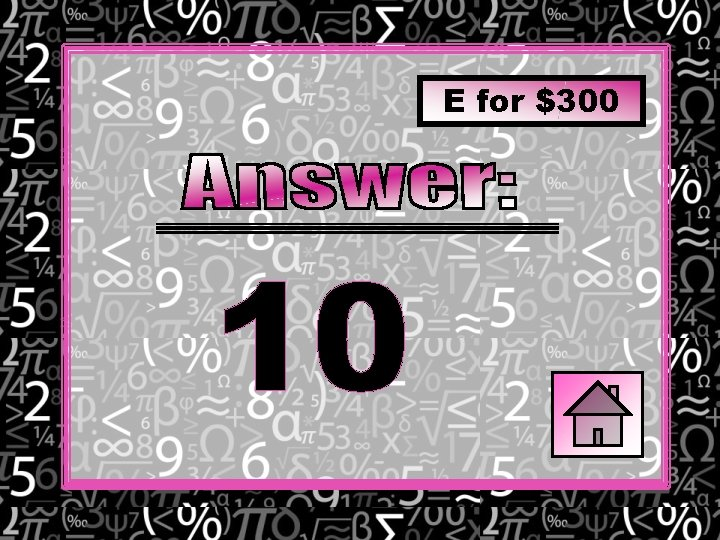E for \$300 10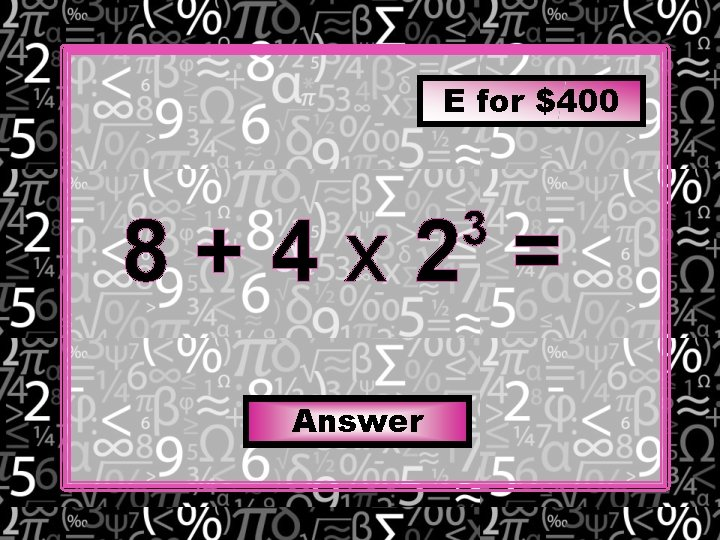E for \$400 8+4 x 2 = 3 AnswerE for \$400 40MD for \$100 5 x 6 3= Answer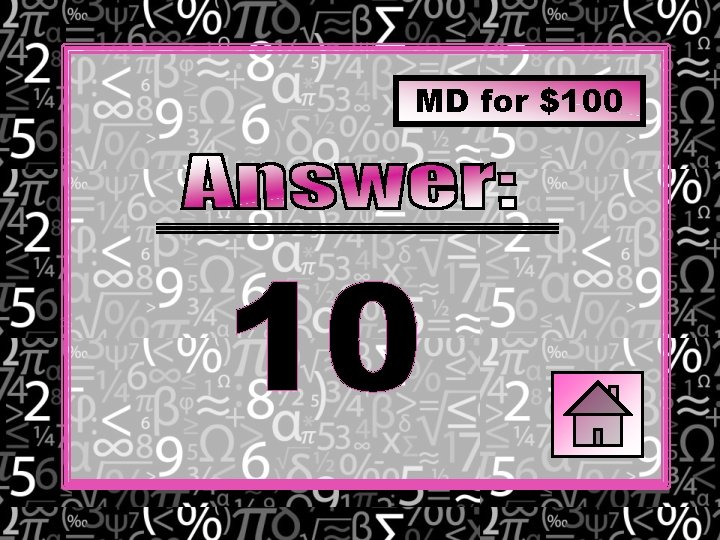MD for \$100 10MD for \$200 30 5 x 2 x 3 = AnswerMD for \$200 36MD for \$300 48 6 x 3 12 = Answer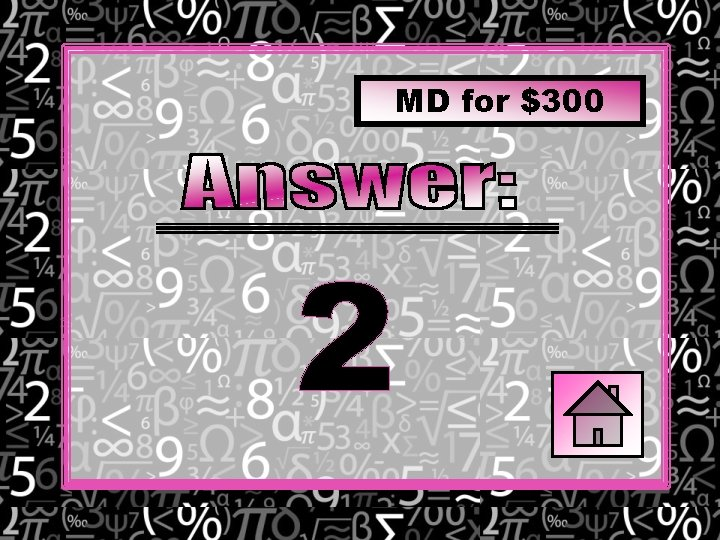MD for \$300 2MD for \$400 6 x 4 8 x 6 2= AnswerMD for \$400 9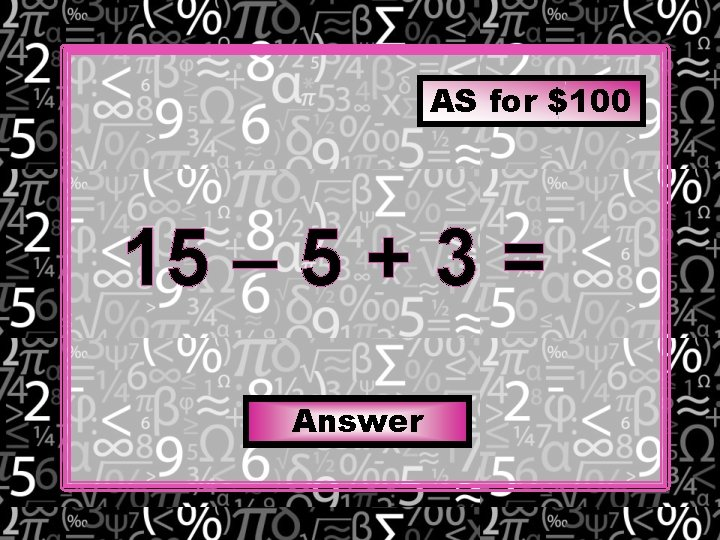AS for \$100 15 – 5 + 3 = Answer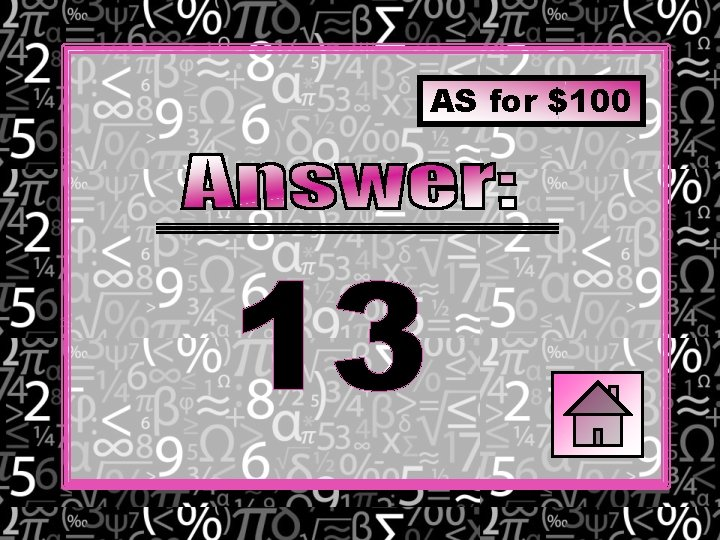AS for \$100 13AS for \$200 2 + 10 – 5 + 9 = Answer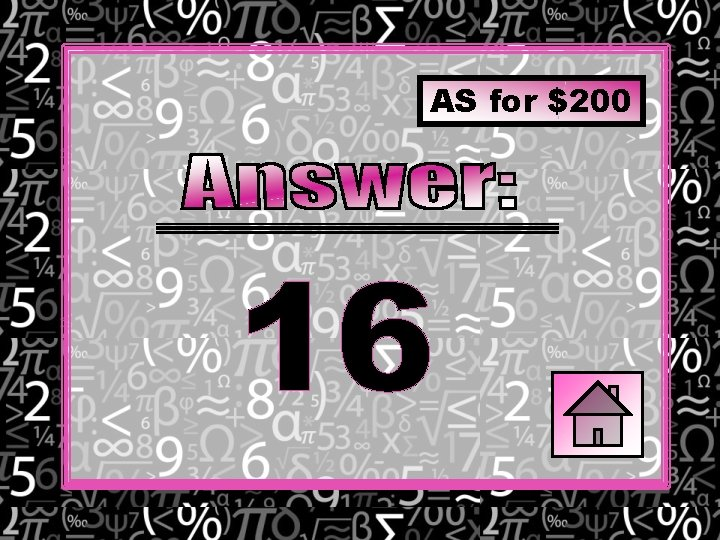AS for \$200 16AS for \$300 15 – 3 + 8 – 5 = Answer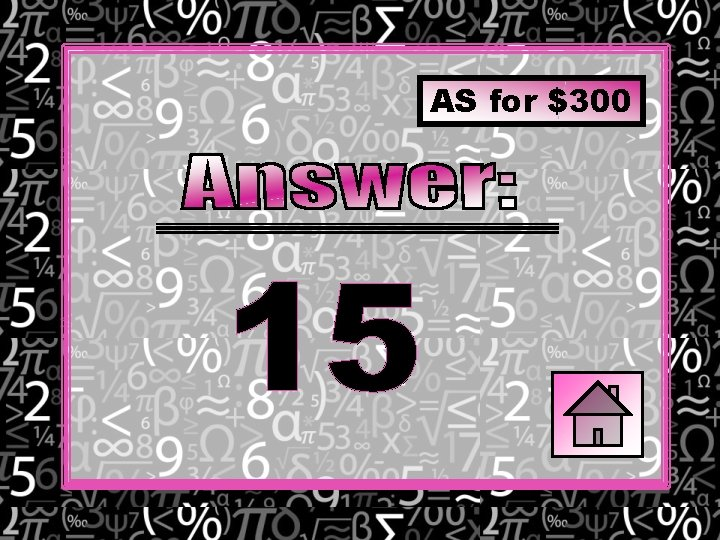AS for \$300 15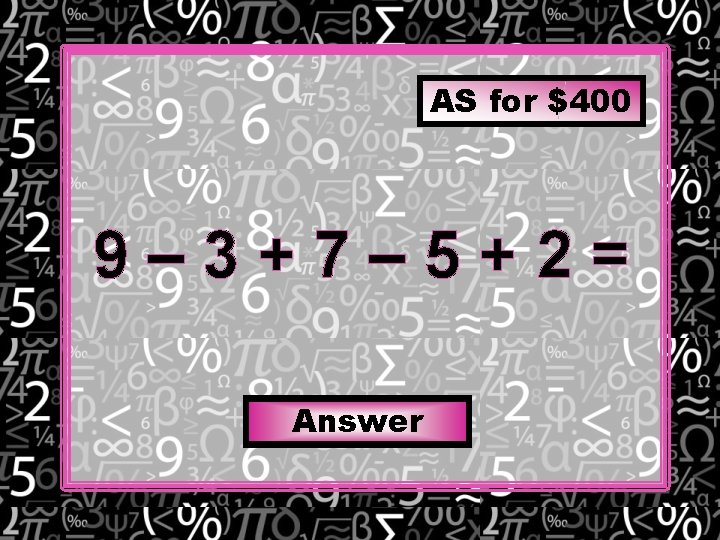AS for \$400 9– 3+7– 5+2= Answer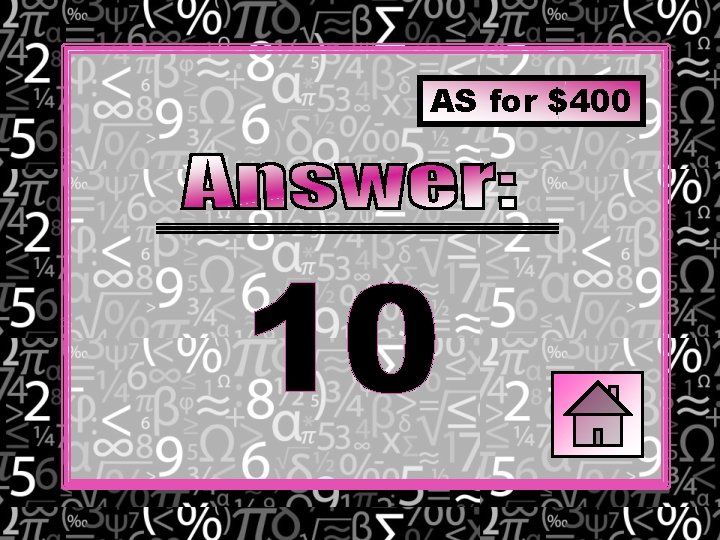AS for \$400 10PEMDAS for \$100 2 3 x 2 (6 – 3) = Answer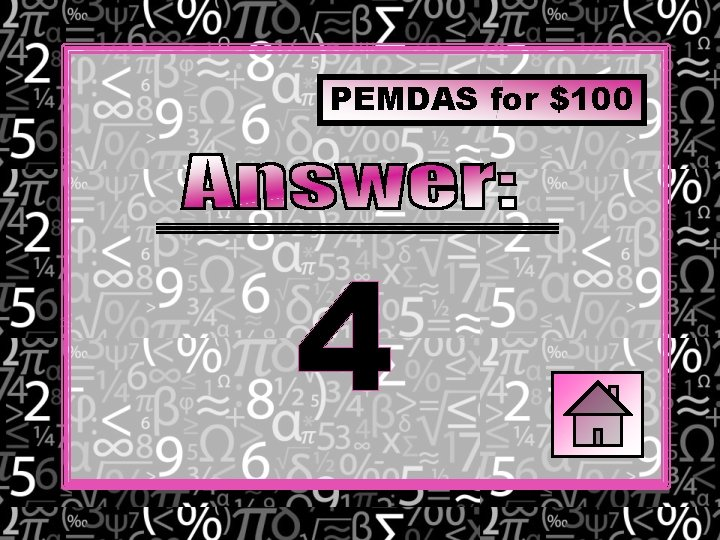PEMDAS for \$100 4PEMDAS for \$200 32 4 + 4 x 3 Answer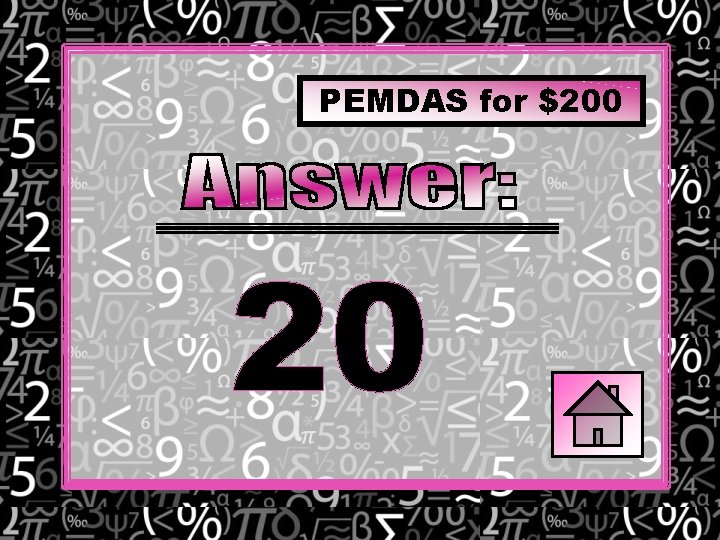PEMDAS for \$200 20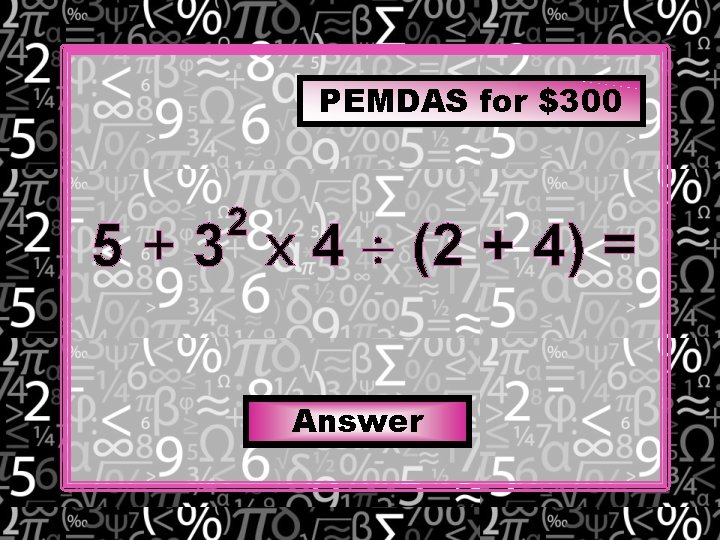PEMDAS for \$300 2 5 + 3 x 4 (2 + 4) = Answer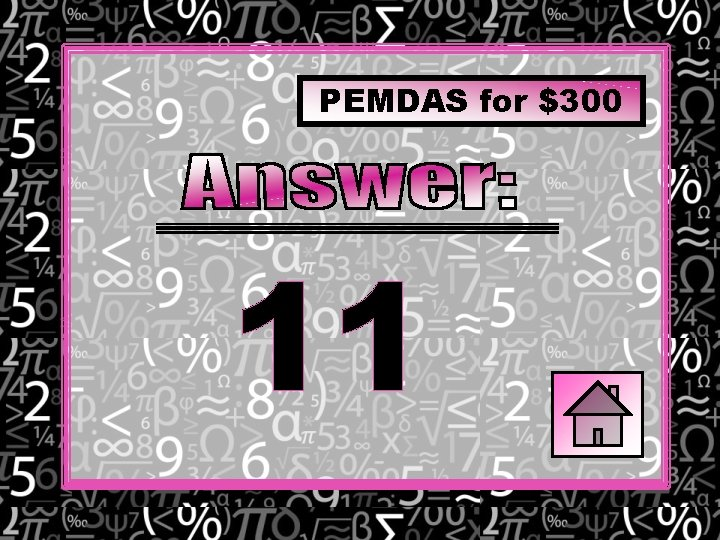PEMDAS for \$300 11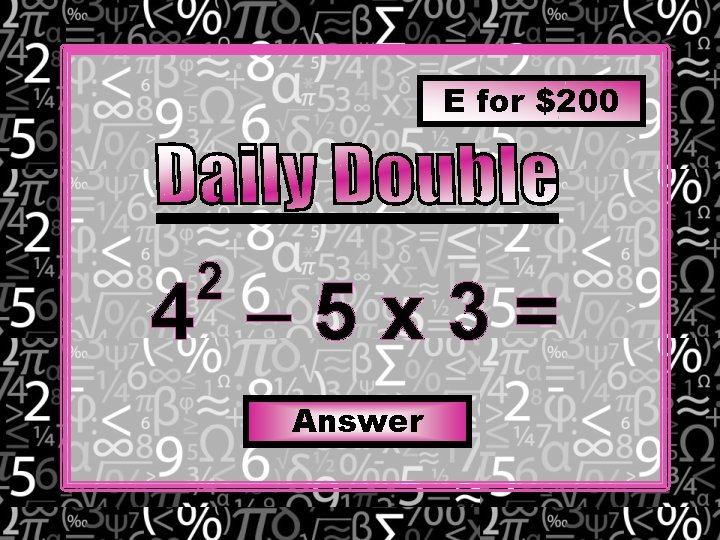E for \$200 2 4 – 5 x 3= AnswerE for \$200 1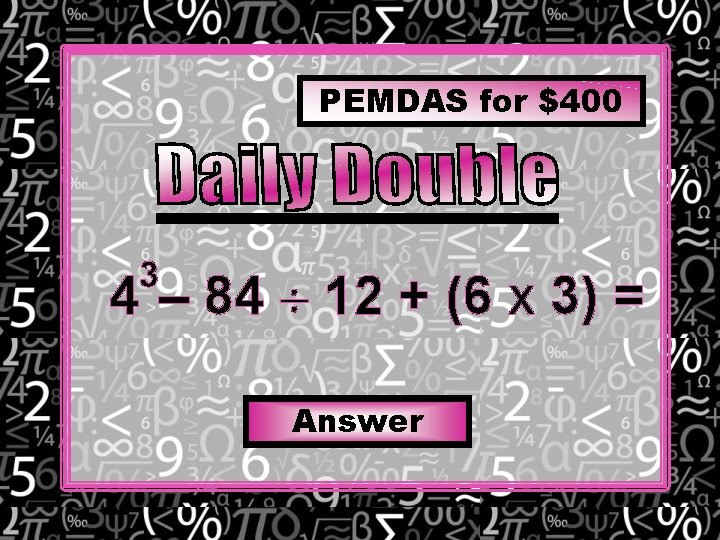PEMDAS for \$400 3 4 – 84 12 + (6 x 3) = Answer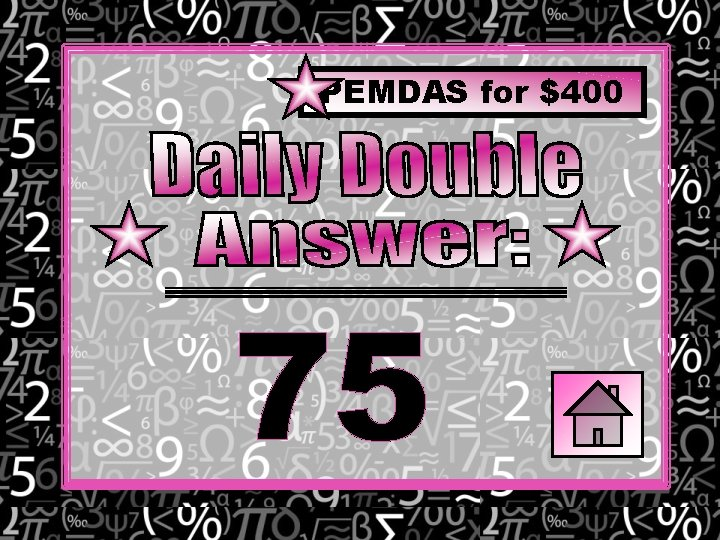PEMDAS for \$400 75P for \$300 (3 +12) – (4 + 2) = AnswerP for \$300 9\$1, 000 4 2 43 + (97 – 3 ) 2 – 5 x 6 = Answer\$1, 000 17Add up the total amount of money you won!!!